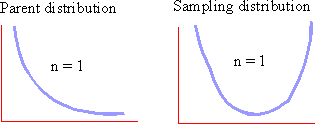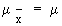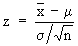Support the Monkey! Tell All your Friends and TeachersHome MonkeyNotes Printable Notes Digital Library Study Guides Study Smart Parents Tips College Planning Test Prep Fun Zone Help / FAQ How to Cite Request a New Title

8.6 Central Limit Theorem

What we have discussed above with an example, is stated as a theorem called Central limit theorem. It states that 'when the size of the sample increases and becomes sufficiently large the distribution of the sample means tends to Normal Distribution'.

Now here we must take one thing in our notice that the theorem is equally valid for both normal and strongly non-normal. We restate the central limit theorem as: "Even if the population distribution is strongly non-normal, its sampling distribution of means tends to normal distribution for large sample size".

The natural question that comes to ones mind is as to what will be the size which can be taken as the large size?

The following diagrams show how sampling distribution of means approaches normal distribution even if the parent distribution is not normal.The answer to the above question is that the "large sample size" means n > 30 i.e. over 30.Your browser does not support the IFRAME tag.

We have seen that the sample meanis distributed normally with mean m , the mean of the population which is an unbiased estimator. It can be shown that the standard deviation of the sample means, called Standard error (S.E.) is equal toThusandIf we put,then z - score is called a standard normal variate, with the mean as zero and the standard deviation as 1. If m is the population mean andis the sample mean then- m is the deviation of the sample mean from the population mean.

When this deviation is divided by, the ratiois called the relative deviation of the sample mean from the population mean.

Index

8.1 Population
8.2 Sample
8.3 Parameters and Statistic
8.4 Sampling Distribution
8.5 Sampling Error
8.6 Central Limit Theorem
8.7 Critical Region
8.8 Testing of Hypothesis
8.9 Errors in Tesitng of Hypothesis
8.10 Power o a Hypothesis Test
8.11 Sampling of Variables
8.12 Sampling of Attributes
8.13 Estimation
8.14 Testing the Difference Between Means
8.15 Test for Difference Between Proportions
8.16 Two Tailed and one Tailed Tests
8.17 Test of Significance for Small Samples
8.18 Students t-distribution
8.19 Distribution of 't' for Comparison of Two Samples Means Independent Samples
8.20 Testing Difference Between Mens of Two Samples Dependent Samples or Matched Paired Observations
8.21 Chi-Square
8.22 Sampling Theory of Correlation
8.23 Sampling Theory of Regression

Chapter 1Search: All Products Books Popular Music Classical Music Video DVD Toys & Games Electronics Software Tools & Hardware Outdoor Living Kitchen & Housewares Camera & Photo Cell Phones Keywords: